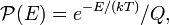# Boltzmann constant

(Redirected from Boltzmann's constant)

The Boltzmann constant k (also kB) is the ratio of the molar gas constant R to Avogadro's constant NA. It can be thought of as the gas constant for a single molecule (or even for an arbitrary particle in a colloidal solution) rather than for a mole.

The constant appears in the Maxwell-Boltzmann distribution:$\mathcal{P}(E) = e^{-E/(kT)}/Q,$

where$\mathcal{P}(E)$ is the probability that a physical system, in thermal equilibrium with absolute temperature T, has energy E. The normalization constant Q is known as a partition function. In classical statistical thermodynamics it is an integral over the phase space (space of positions and momenta of the particles).

The Boltzmann constant appears also in the equation for the translational kinetic energy of a simple particle in thermal equilibrium with its surroundings:$KE_\mathrm{avg} = \left(\tfrac{3}{2}\right) kT$

Where KEavg is the average kinetic energy of the particle, k is the Boltzmann constant, and T is the temperature in kelvin.

According to NIST the Boltzmann constant has a value of 1.3806504 × 10−23 J/K with a standard uncertainty of 0.0000024 × 10−23 J/K and a relative uncertainty of 1.7 × 10−6 (this is represented by the concise form 1.380 6504(24) × 10−23 J/K

The Boltzmann constant can also be represented in alternative units as 8.617385 × 10−5 eV/K